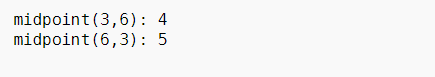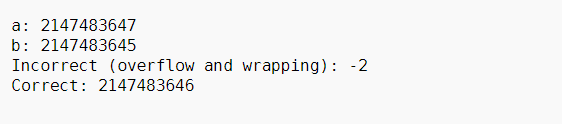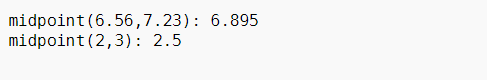midpoint() in C++20 with Examples

• Last Updated : 27 Aug, 2021

The function midpoint() computes the midpoint of the integers, floating-points, or pointers a and b.

<numeric>

Parameters: This function accepts two data types like integers, floating points, pointer values.

Return:

It returns the midpoints of the given data types.
Algorithm this function implements:

• Half the sum of a and b without any overflow. Same as, (a + b)/2.
• If a and b are integer types and the sum is odd, the result is rounded towards a.
• If a and b are floating-point types, at most one inexact operation occurs (rounded towards zero).
• If a and b are pointing to x[i] and x[j] respectively of the same array object x (for the purpose of pointer arithmetic), the result will be a pointer to x[i + (j – i)/2] (or, equivalently x[std::midpoint(i, j)]) where the division rounds towards zero. If a and b do not point to elements of the same array object, the behavior is undefined.

Uses Of midpoint() function:

• Middle point of two integers using midpoint(): Below is the C++ Program to demonstrate the middle point of two integer numbers using midpoint():

C++

 // C++ program to demonstrate the // midpoint function #include #include using namespace std;   // Driver Code int main() {     // a and b both of integer type     int a = 3;     int b = 6;       cout << "midpoint(" << a << ", "          << b << "): "          << midpoint(a, b) << endl;       a = 6;     b = 3;     cout << "midpoint(" << a          << ", " << b << "): "          << midpoint(a, b) << endl;       return 0; }

Output:• midpoint() can handle cases like an overflow: Below is the C++ program to demonstrate how to handle overflow using midpoint():

C++

 // C++ program for the above approach #include #include #include using namespace std;   // Driver Code int main() {     // a stores maximum storable     // value of integer     int a = INT_MAX;       // b stores maximum storable     // value of integer - 2     int b = INT_MAX - 2;       cout << "a: " << a << endl          << "b: " << b << endl          << "Incorrect (overflow"          << " and wrapping): "          << (a + b) / 2 << endl          << "Correct: "          << midpoint(a, b) << "\n\n";       return 0; }

Output:• Middle point of two floating number using midpoint(): Below is the C++ program to demonstrate how to find the middle point of two floating-point numbers using midpoint():

C++

 // C++ program for the above approach #include #include   using namespace std;   int main() {     // x and y both floating type     float x = 6.56;     float y = 7.23;       cout << "midpoint(" << x          << ", " << y << "): "          << midpoint(x, y) << endl;       x = 2.0;     y = 3.0;       cout << "midpoint(" << x          << ", " << y << "): "          << midpoint(x, y)          << endl          << endl; }

Output:• Middle point between two pointers pointing to the same object using midpoint(): Below is the C++ program to demonstrate how to find the middle point of two pointers pointing to the same object using midpoint():

C++

 // C++ program for the above approach #include #include using namespace std;   // Driver Code int main() {     // str is a character array     char str[] = "GeeksforGeeks";       // str1 is pointing to the     // 5-th element in str     char* str1 = &str;       // str2 is pointing to the     // 10-th element in str     char* str2 = &str;       // str1 and str2 is pointing same     // object str therefore, we can     // logically compute and point a     // middle element in str     cout << "midpoint('" << *str1          << "', '" << *str2 << "'): '"          << *midpoint(str1, str2)          << "'" << endl;       cout << "midpoint('" << *str2          << "', '" << *str1 << "'): '"          << *midpoint(str2, str1)          << "'" << endl; }

Output:Want to learn from the best curated videos and practice problems, check out the C++ Foundation Course for Basic to Advanced C++ and C++ STL Course for foundation plus STL.  To complete your preparation from learning a language to DS Algo and many more,  please refer Complete Interview Preparation Course.

My Personal Notes arrow_drop_up
Recommended Articles
Page :